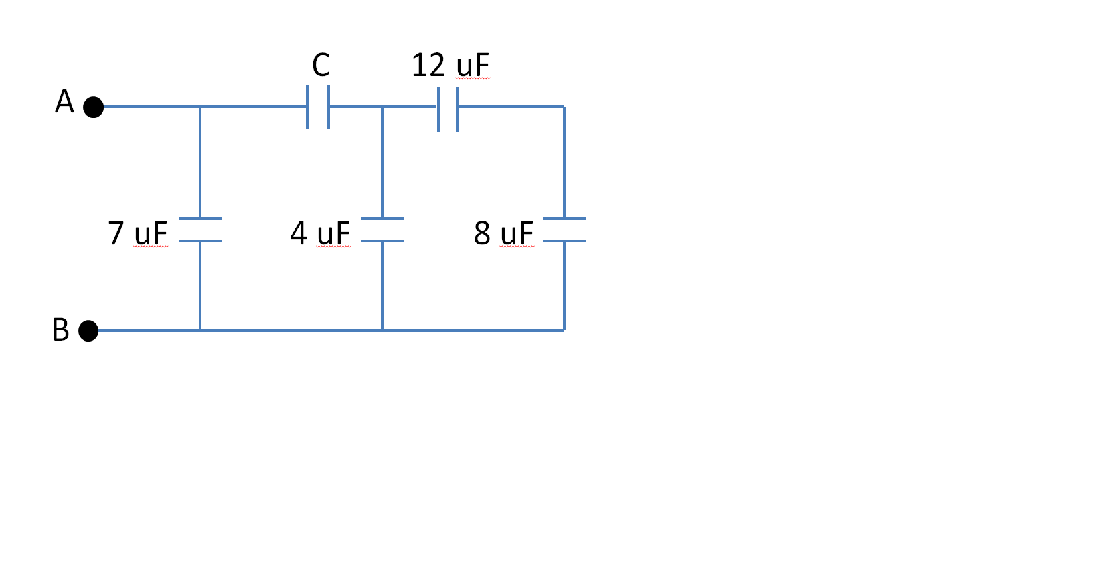Problem: Consider the circuit of capacitors shown below. The equivalent capacitance of the circuit is 9.0 μF.Determine the value of the capacitance C.

FREE Expert Solution
95% (225 ratings)
Problem Details

Consider the circuit of capacitors shown below. The equivalent capacitance of the circuit is 9.0 μF.

Determine the value of the capacitance C.